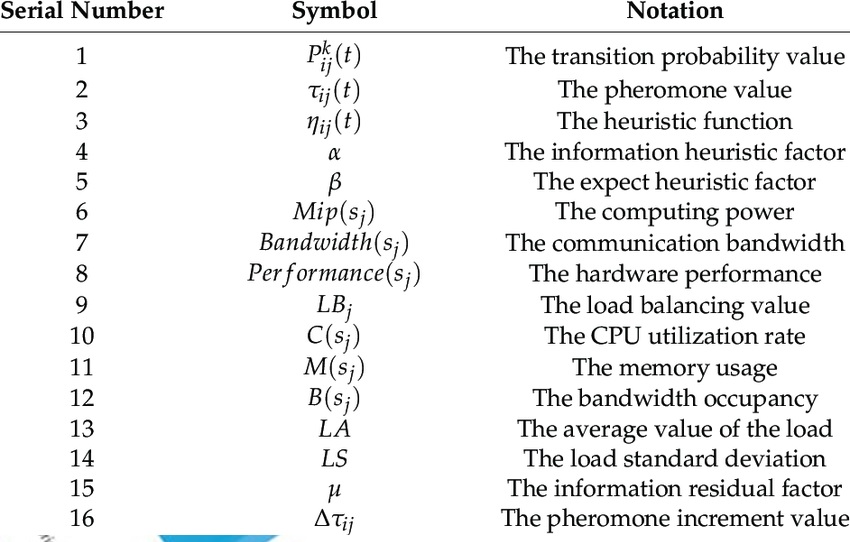##### Latest NewsIn Mathematics, the mathematical notation is defined as the symbolic representation of the ideas and objects. The notations are used in different fields like Physics, Engineering, Economics, and other fields to precisely understand the concept statement. It is a system of writing expressions which has a valuable semantic meaning. We know that Mathematics is a universal language, and everyone understands it. It is due to the symbols present in the expression. Mathematical expressions consist of a sequence of numbers, and operators, that can be evaluated. We have many symbols in Mathematics, and the concept of Maths is entirely dependent on the symbols. Most of the symbols have predefined values, which makes it easy to evaluate the expression quickly.

There are various symbols in Maths, starting from simple addition to the complex integration symbols. There are many equality and inequality symbols used in the stream of mathematics, which clearly explains the concept behind the mathematical idea. For example, if we want to arrange the numbers in the ascending order, it can be represented using the less than symbol. 2, 3, 4, 5, 6, 7 are the numbers arranged from the smallest to the largest order. It can be symbolically represented as 2<3<4<5<6<7. Both represent the concept of ascending order. It is essential to get a complete knowledge of Maths symbols to solve the problems efficiently. We have many symbols in Maths such as basic symbols, logical symbols, roman numerals, calculus symbols, and so on. Mathematicians commonly use the Greek alphabets to represent the constant, variables, functions, etc. So, learning different mathematical symbols is a vital part of language development and mathematical proficiency. Now, let us discuss some of the advantages of Maths symbols.

## Advantages of Using Mathematical Notations

Some of the advantages of using Mathematical symbols are:

### Helps to Represent Quantities

In Algebra, many mathematical notations are used to represent the quantities or numbers. To solve mathematical problems, we need variables and constants. We can use the variables as the placeholder to represent the unknown quantities.

### Helps to Identify the Operation Type

We have different types of operations in Maths, such as addition, subtraction, multiplication and division, integration, differentiation, and so on. By using the notations in Maths, we can easily identify the types of operations involved in the expression. For example, 5+12+3 = 20. It is an arithmetic equation, which contains the “+” symbol. So, the operation involved here is “Addition”.

### Establishes the Relationship between Quantities

Many mathematical notions are used to express the relationship between two quantities. For example, Ram is older than Rahul. It is clearly understood that the age of Ram is greater than Rahul. It is mathematically represented using the greater than symbol. Now, let us take the age of Ram to be “x” and the age of Rahul to be “y”. The expression to represent this is “x>y”.

### Breaks the Language Barrier

The various notations used in Mathematics are globally applicable, and it breaks the language barrier. The symbols are considered as a useful tool for global communication and understanding. For example, 2+3= 5 is a symbolic representation of the statement “The addition/sum of 2 and 3 is 5”. So, it is understood that different mathematical symbols break the language barrier.